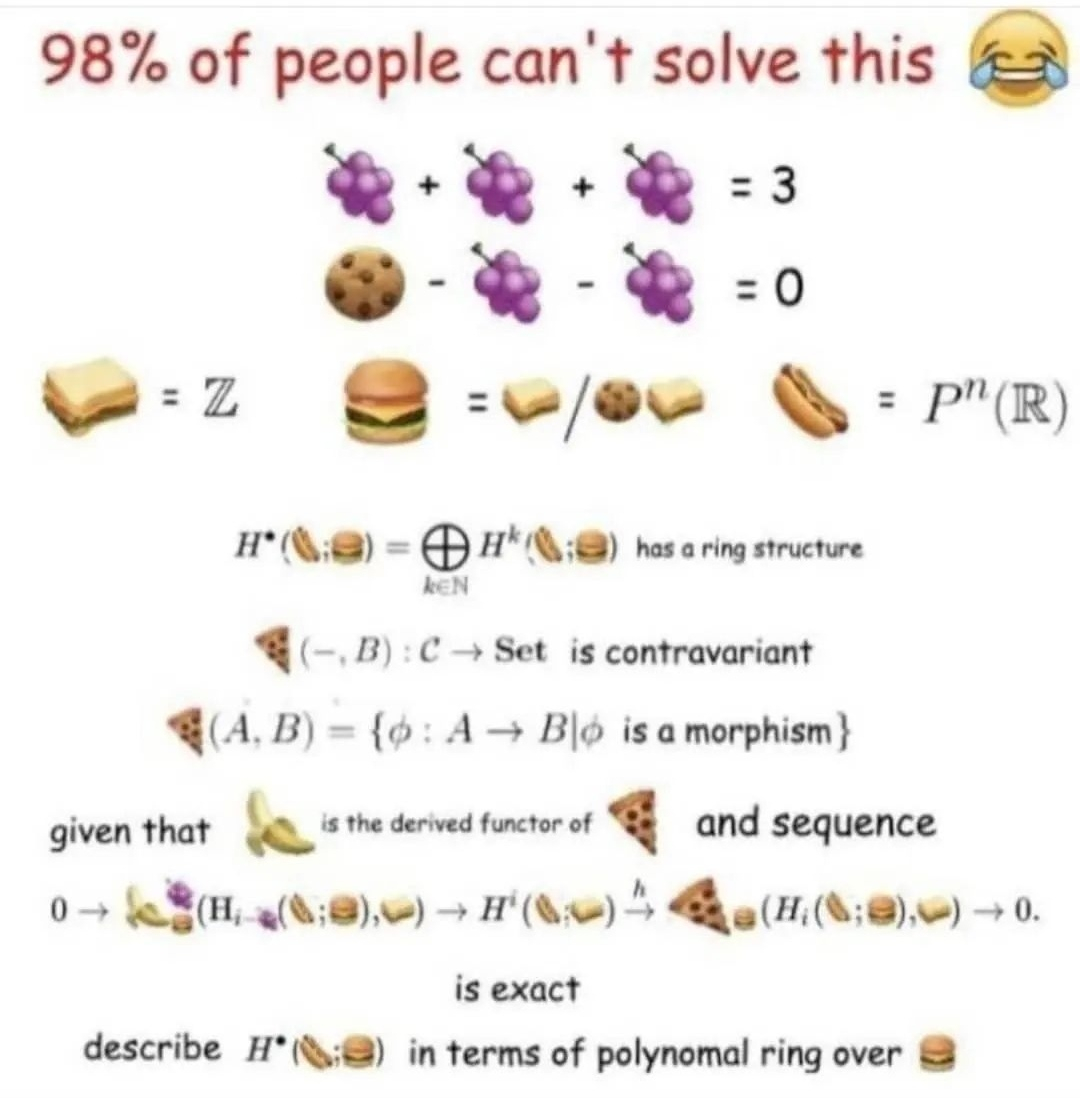#### Howdy, Stranger!

It looks like you're new here. Sign in or register to get started.Attention: Please take a moment to consider our terms and conditions before posting.

# 230 - 220 x 0.5 = 5!

• Correct. Nothing to see here, move on.
• (230-220) x 0.5
10 halved (as 0.5 is half a whole number)
10 x 0.5 = 5
• One point to bob, one point off johnny
• bobmunro said:
230 - (220 x 0.5) = 120

5! = 5x4x3x2x1 = 120

QED
This

• Am sure we have had multiple bidmas/Bodmas type questions on here before
• I think we’ve had this exact one before.

5! not 5 is what makes it correct.
• to explain 5factorial (5!) in more detail
5! = 5 × 4 × 3 × 2 × 1 = 120

• My trusty old scientific calculator which has got me through 12/13 accounts exams to date says 120!
• 120. I’m sure we had a thread like this before. That’s the only way I concluded it’s 120 rather than 5.
• BODMAS
Exactly  !

Need to know what part of the equation to do first. If you take it at face value then the answer is 5. But put a couple of brackets in & it would change the answer completely.

Edit.

No idea what an exclamation mark at the end means - never used it when I was at school in the early 80's but then I only did "O" level maths. I just thought Chizz was exclaiming the answer.
• I think we’ve had this exact one before.

5! not 5 is what makes it correct.
But why do you have to say factorial to make it correct ?
The question is not 'what is the factorial of this equation'
• MrOneLung said:
I think we’ve had this exact one before.

5! not 5 is what makes it correct.
But why do you have to say factorial to make it correct ?
The question is not 'what is the factorial of this equation'
The only way you can solve the problem using BODMAS principles(or rather agree the answer) is by recognising the exclamation mark as the mathematical symbol for Factorial rather than, as many would, see the exclamation mark as indicating a surprising answer.
• bobmunro said:
MrOneLung said:
I think we’ve had this exact one before.

5! not 5 is what makes it correct.
But why do you have to say factorial to make it correct ?
The question is not 'what is the factorial of this equation'
The only way you can solve the problem using BODMAS principles(or rather agree the answer) is by recognising the exclamation mark as the mathematical symbol for Factorial rather than, as many would, see the exclamation mark as indicating a surprising answer.
I didn't even see the ! on the original question - I thought people were just saying the answer was 120 and then taking it further to say that is 5!
• BODMAS

I was taught it as BIMDAS; Brackets, Indices, Multiplication/Division, Addition/Subtraction.
• BODMAS

I was taught it as BIMDAS; Brackets, Indices, Multiplication/Division, Addition/Subtraction.
Either is common - O (Orders) is probable better as it covers powers, roots and indices.

• Listen lads stand aside i'm a maths teacher...

6ft 2inches

I didn't say i was a good maths teacher!
• I think we’ve had this exact one before.

5! not 5 is what makes it correct.
And here's me thinking 5! is five said very forcefully.
•The bloke who created the theory behind this died at 20 so shouldn't be too difficult.
• Chizz said:
Wrong.
How so?
order of operations convention designed to overcome unintentional ambiguity.  230 - 220 x 0.5 is calculated as 230 - (220x0.5), not (230-220) x 0.5.  If the latter is what is wanted that is the form in which it would need to be written.

Edit - I am wrong.  5! = 120.  Thank-you for introducing me to factorials.  If I'd bothered to read the thread I'd have discovered this by post 4.

I've been sums shamed.